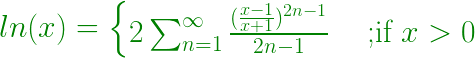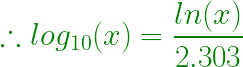# Find the ln(X) and log10X with the help of expansion

• Difficulty Level : Medium
• Last Updated : 11 May, 2021

Given a positive number x, the task is to find the natural log (ln) and log to the base 10 (log10) of this number with the help of expansion.

Example:

Input: x = 5
Output: ln 5.000 = 1.609
log10 5.000 = 0.699

Input: x = 10
Output: ln 10.000 = 2.303
log10 10.000 = 1.000

Approach:

• The expansion of natural logarithm of x (ln x) is:

• Therefore this series can be summed up as:• Hence a function can be made to evaluate the nth term of the sequence for 1 ≤ x ≤ n
• Now to calculate log10 x, below formula can be used:Below is the implementation of the above approach:

## C++

 // CPP code to Find the ln x and// log10 x with the help of expansion #include #include #include  using namespace std; // Function to calculate ln x using expansiondouble calculateLnx(double n){     double num, mul, cal, sum = 0;    num = (n - 1) / (n + 1);     // terminating value of the loop    // can be increased to improve the precision    for (int i = 1; i <= 1000; i++) {        mul = (2 * i) - 1;        cal = pow(num, mul);        cal = cal / mul;        sum = sum + cal;    }    sum = 2 * sum;    return sum;} // Function to calculate log10 xdouble calculateLogx(double lnx){    return (lnx / 2.303);} // Driver Codeint main(){     double lnx, logx, n = 5;    lnx = calculateLnx(n);    logx = calculateLogx(lnx);     // setprecision(3) is used to display    // the output up to 3 decimal places     cout << fixed << setprecision(3)         << "ln " << n << " = "         << lnx << endl;    cout << fixed << setprecision(3)         << "log10 " << n << " = "         << logx << endl;}

## Java

 // Java code to Find the ln x and// log10 x with the help of expansionimport java.io.*; class GFG{     // Function to calculate ln x using expansionstatic double calculateLnx(double n){    double num, mul, cal, sum = 0;    num = (n - 1) / (n + 1);     // terminating value of the loop    // can be increased to improve the precision    for (int i = 1; i <= 1000; i++)    {        mul = (2 * i) - 1;        cal = Math.pow(num, mul);        cal = cal / mul;        sum = sum + cal;    }    sum = 2 * sum;    return sum;} // Function to calculate log10 xstatic double calculateLogx(double lnx){    return (lnx / 2.303);} // Driver Codepublic static void main (String[] args){    double lnx, logx, n = 5;    lnx = calculateLnx(n);    logx = calculateLogx(lnx);         // setprecision(3) is used to display    // the output up to 3 decimal places         System.out.println ("ln " + n + " = " + lnx );    System.out.println ("log10 " + n + " = "+ logx );}} // This code is contributed by ajit

## Python3

 # Python 3 code to Find the ln x and# log10 x with the help of expansion# Function to calculate ln x using expansionfrom math import powdef calculateLnx(n):    sum = 0    num = (n - 1) / (n + 1)     # terminating value of the loop    # can be increased to improve the precision    for i in range(1, 1001, 1):        mul = (2 * i) - 1        cal = pow(num, mul)        cal = cal / mul        sum = sum + cal     sum = 2 * sum    return sum # Function to calculate log10 xdef calculateLogx(lnx):    return (lnx / 2.303) # Driver Codeif __name__ == '__main__':    n = 5    lnx = calculateLnx(n)    logx = calculateLogx(lnx)     # setprecision(3) is used to display    # the output up to 3 decimal places     print("ln", "{0:.3f}".format(n),           "=", "{0:.3f}".format(lnx))    print("log10", "{0:.3f}".format(n),              "=", "{0:.3f}".format(logx))     # This code is contributed by# Surendra_Gangwar

## C#

 // C# code to Find the ln x and// log10 x with the help of expansionusing System;     class GFG{     // Function to calculate ln x using expansionstatic double calculateLnx(double n){    double num, mul, cal, sum = 0;    num = (n - 1) / (n + 1);     // terminating value of the loop    // can be increased to improve the precision    for (int i = 1; i <= 1000; i++)    {        mul = (2 * i) - 1;        cal = Math.Pow(num, mul);        cal = cal / mul;        sum = sum + cal;    }    sum = 2 * sum;    return sum;} // Function to calculate log10 xstatic double calculateLogx(double lnx){    return (lnx / 2.303);} // Driver Codepublic static void Main (String[] args){    double lnx, logx, n = 5;    lnx = calculateLnx(n);    logx = calculateLogx(lnx);         // setprecision(3) is used to display    // the output up to 3 decimal places         Console.WriteLine("ln " + n + " = " + lnx );    Console.WriteLine("log10 " + n + " = "+ logx );}} // This code is contributed by Princi Singh

## Javascript

 
Output:
ln 5.000 = 1.609
log10 5.000 = 0.699

My Personal Notes arrow_drop_up